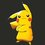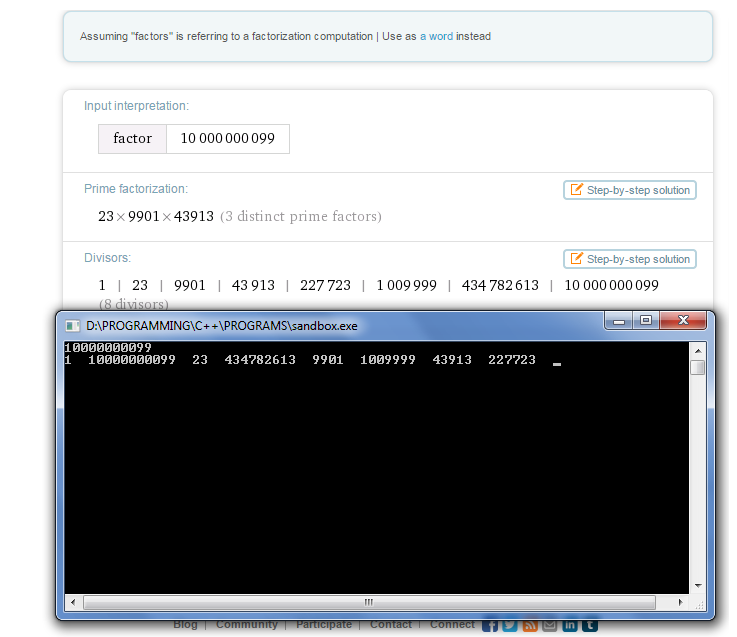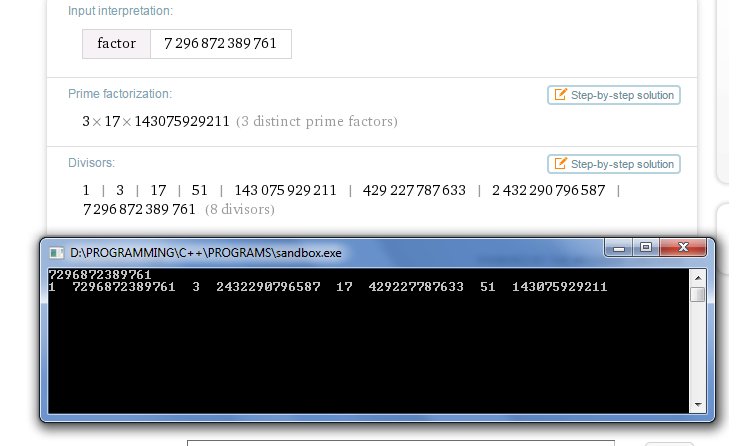# Factorizing in C++

Can anyone help me out in improving the following program to find factors of number $n$ as it doesn't work for higher numbers.

  1 2 3 4 5 6 7 8 9 10 11 12 13 14 15 #include #include void main() { clrscr(); cin>>n; for (int a=1;aNote by Pranjal Jain
6 years, 1 month ago

This discussion board is a place to discuss our Daily Challenges and the math and science related to those challenges. Explanations are more than just a solution — they should explain the steps and thinking strategies that you used to obtain the solution. Comments should further the discussion of math and science.

When posting on Brilliant:

• Use the emojis to react to an explanation, whether you're congratulating a job well done , or just really confused .
• Ask specific questions about the challenge or the steps in somebody's explanation. Well-posed questions can add a lot to the discussion, but posting "I don't understand!" doesn't help anyone.
• Try to contribute something new to the discussion, whether it is an extension, generalization or other idea related to the challenge.
• Stay on topic — we're all here to learn more about math and science, not to hear about your favorite get-rich-quick scheme or current world events.

MarkdownAppears as
*italics* or _italics_ italics
**bold** or __bold__ bold
- bulleted- list
• bulleted
• list
1. numbered2. list
1. numbered
2. list
Note: you must add a full line of space before and after lists for them to show up correctly
paragraph 1paragraph 2

paragraph 1

paragraph 2

[example link](https://brilliant.org)example link
> This is a quote
This is a quote
    # I indented these lines
# 4 spaces, and now they show
# up as a code block.

print "hello world"
# I indented these lines
# 4 spaces, and now they show
# up as a code block.

print "hello world"
MathAppears as
Remember to wrap math in $$ ... $$ or $ ... $ to ensure proper formatting.
2 \times 3 $2 \times 3$
2^{34} $2^{34}$
a_{i-1} $a_{i-1}$
\frac{2}{3} $\frac{2}{3}$
\sqrt{2} $\sqrt{2}$
\sum_{i=1}^3 $\sum_{i=1}^3$
\sin \theta $\sin \theta$
\boxed{123} $\boxed{123}$

## Comments

Sort by:

Top Newest

How many times should i try to help before someone notices Pranjal Jain . I have included a few ideas in the following from Sudeep Salgia as well:

  1 2 3 4 5 6 7 8 9 10 11 12 13 14 15 16 17 #include #include #include int main() { double m,i,k=10; cin>>m; for(i=1;i

Here are the factors of $10000000099$And here are the factors of $7296872389761$- 6 years ago

Log in to reply

Thanks. Sorry for not noticing. I am quite inactive now a days on B'ant. (Turned off all email notifications as well)

- 6 years ago

Log in to reply

- 6 years ago

Log in to reply

Try long double it has the max and min values $10^{4932}\quad and \quad 10^{-4932}$ respectively and has a memory of 10 bytes. Its the biggest as far as I know.

- 6 years, 1 month ago

Log in to reply

Modulus doesn't work with floating data types.

- 6 years, 1 month ago

Log in to reply

You can firstly improve by running the loop till $i \leq \frac{n}{2}$. Or you can try this:

 1 2 3 4 5 6 7 8 9 int n; cin>>n; for(int l=1, int h=n ; l<=h ; l++,h=n/l) { if(n%l==0) { cout<

- 6 years, 1 month ago

Log in to reply

Yes, I noticed $\frac{n}{2}$ thing. Can you explain algorithm you used? Thanks.

- 6 years, 1 month ago

Log in to reply

So basically we can pair up the divisors for any given $n$. So, l will have the smaller one and h will store the larger one ( such that $l \times h =n$ ) and we are incrementing l by one each time and correspondingly decreasing h. So h will decrease much faster and we would be skipping lot of numbers which anyway we need not check.

PS: I forgot to include the line to check whether $l= h$ that is if n is a perfect square.

- 6 years ago

Log in to reply

It showed many errors. Which compiler are you using? And I believe the condition should be if (n%h==0)

- 6 years ago

Log in to reply

We can bring down $\frac{n}{2}$ to $\lfloor\sqrt{n}\rfloor$. I still can't understand how will it help while dealing with higher numbers. Let me compile.

- 6 years ago

Log in to reply

Maybe you could try using long variable or long long . But that won't be really necessary if you are writing this for boards

- 6 years, 1 month ago

Log in to reply

I program for fun. -_- I hate boards

- 6 years, 1 month ago

Log in to reply

Oh ! So have you studied any algorithm books like CLRS ? Or are you active on websites like topcoder , spoj ?

- 6 years, 1 month ago

Log in to reply

Currently I am inactive due to JEE. But I will be active after 2-3 months on Project Euler.

- 6 years ago

Log in to reply

Try Java Codes,They are always better than C++

- 6 years, 1 month ago

Log in to reply

For that, I'll have to learn java properly.

- 6 years, 1 month ago

Log in to reply

Try this out

 1 2 3 4 5 6 7 8 double n,i; cin>>n; for(i=1;i<=(n/2);i++) { double k=floor(n/i); if (i*k==n) cout<

- 6 years, 1 month ago

Log in to reply

Why are you taking

 1 2 Double k=floor(n/i); If(i*k==n) 

to check if $i$ is a factor of $n$ instead of

 1 If(n%i == 0) 

- 6 years, 1 month ago

Log in to reply

Big numbers cannot be stored in long. Therefore we need something which is even bigger, like float or double. The problem with using "%" function with float/double is that it will not work. "%" is meant to work only with integers(int or long or short), It will not work with float and double as they are decimal values. Does 100%2.3 make sense?

Hence we use a workaround to find the true factors of n by using floor.

- 6 years, 1 month ago

Log in to reply

Ouch. I didn't notice that you used double. But then why not use long long? long long has a higher range than a double for the same memory, since a double can only hold 15 significant digits whereas long long can hold upto 18.

Note I don't use C++ so I have no idea if long long is used commonly.

- 6 years, 1 month ago

Log in to reply

Nope, long long doesn't work in c++. That's the biggest problem here.

- 6 years, 1 month ago

Log in to reply

×

Problem Loading...

Note Loading...

Set Loading...